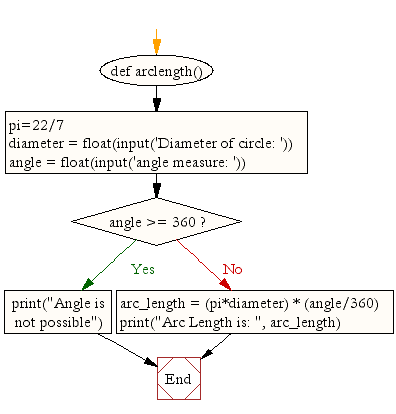﻿ Python Math: Calculate arc length of an angle - w3resource# Python Math: Calculate arc length of an angle

## Python Math: Exercise-7 with Solution

Write a Python program to calculate arc length of an angle.
Note: In a planar geometry, an angle is the figure formed by two rays, called the sides of the angle, sharing a common endpoint, called the vertex of the angle. Angles formed by two rays lie in a plane, but this plane does not have to be a Euclidean plane.

Sample Solution:-

Python Code:

``````def arclength():
pi=22/7
diameter = float(input('Diameter of circle: '))
angle = float(input('angle measure: '))
if angle >= 360:
print("Angle is not possible")
return
arc_length = (pi*diameter) * (angle/360)
print("Arc Length is: ", arc_length)

arclength()
```
```

Sample Output:

```Diameter of circle: 9
angle measure: 45
Arc Length is:  3.5357142857142856
```

Pictorial Presentation:Flowchart:## Visualize Python code execution:

The following tool visualize what the computer is doing step-by-step as it executes the said program:

Python Code Editor:

Have another way to solve this solution? Contribute your code (and comments) through Disqus.

What is the difficulty level of this exercise?

Test your Programming skills with w3resource's quiz.

﻿

## Python: Tips of the Day

Difference between staticmethod and classmethod:

Maybe a bit of example code will help: Notice the difference in the call signatures of foo, class_foo and static_foo:

```class A(object):
def foo(self, x):
print "executing foo(%s, %s)" % (self, x)

@classmethod
def class_foo(cls, x):
print "executing class_foo(%s, %s)" % (cls, x)

@staticmethod
def static_foo(x):
print "executing static_foo(%s)" % x

a = A()
```

Below is the usual way an object instance calls a method. The object instance, a, is implicitly passed as the first argument.

```a.foo(1)
# executing foo(<__main__.A object at 0xb7dbef0c>,1)
```

With classmethods The class of the object instance is implicitly passed as the first argument instead of self.

```a.class_foo(1)
# executing class_foo(<class '__main__.A'>,1)
```

You can also call class_foo using the class. In fact, if you define something to be a classmethod, it is probably because you intend to call it from the class rather than from a class instance. A.foo(1) would have raised a TypeError, but A.class_foo(1) works just fine:

```A.class_foo(1)
# executing class_foo(<class '__main__.A'>,1)
```

One use people have found for class methods is to create inheritable alternative constructors.

With staticmethods neither self (the object instance) nor cls (the class) is implicitly passed as the first argument. They behave like plain functions except that you can call them from an instance or the class:

```a.static_foo(1)
# executing static_foo(1)

A.static_foo('hi')
# executing static_foo(hi)
```

Staticmethods are used to group functions which have some logical connection with a class to the class.

foo is just a function, but when you call a.foo you don't just get the function, you get a "partially applied" version of the function with the object instance a bound as the first argument to the function. foo expects 2 arguments, while a.foo only expects 1 argument.

a is bound to foo. That is what is meant by the term "bound" below:

```print(a.foo)
# <bound method A.foo of <__main__.A object at 0xb7d52f0c>>
```

With a.class_foo, a is not bound to class_foo, rather the class A is bound to class_foo.

```print(a.class_foo)
# <bound method type.class_foo of <class '__main__.A'>>
```

Here, with a staticmethod, even though it is a method, a.static_foo just returns a good 'ole function with no arguments bound. static_foo expects 1 argument, and a.static_foo expects 1 argument too.

```print(a.static_foo)
# <function static_foo at 0xb7d479cc>
```

And of course the same thing happens when you call static_foo with the class A instead.

```print(A.static_foo)
# <function static_foo at 0xb7d479cc>
```

Ref: https://bit.ly/2XFGOg6